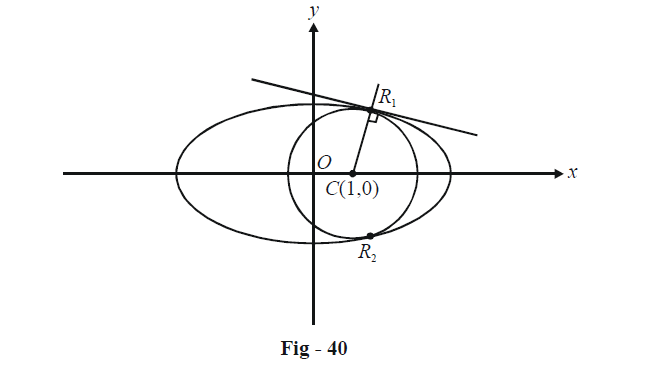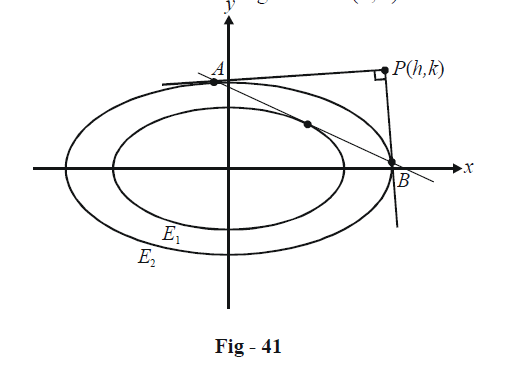# Ellipses Set-2

Go back to  'SOLVED EXAMPLES'

Example – 3

Find the radius of the largest circle with centre (1, 0) that can be inscribed inside the ellipse \begin{align}\frac{{{x^2}}}{{16}} + \frac{{{y^2}}}{4} = 1.\end{align}

Solution: The following diagram shows the largest such circle. Observe it carefully :Note that the largest possible circle lying completely inside the ellipse must touch it, say, at the points R1 and R2, as shown. At these points, it will be possible to draw common tangents to the circle and the ellipse.

Let point R1 be$$(4\cos \theta ,\;\;2\sin \theta ).$$ The equation of the tangent at R1 is

\begin{align}&\qquad\frac{{x\cos \theta }}{4} + \frac{{y\sin \theta }}{2} = 1\\& \Rightarrow\quad x\cos \theta + 2y\sin \theta = 4\end{align}

If CR1 is perpendicular to this tangent ( which must happen if this tangent is to be common to both the ellipse and the circle), we have

\begin{align}&\frac{{2\sin \theta - 0}}{{4\cos \theta - 1}} \times \frac{{ - \cos \theta }}{{2\sin \theta }} = - 1\\ & \Rightarrow\quad \cos \theta = \frac{1}{3}\end{align}

Thus, R1 is $$\left( {\frac{4}{3},\,\,\frac{{4\sqrt 2 }}{3}} \right).$$ The largest possible radius is therefore

\begin{align}&{r_{\max }} = C{R_1} = \sqrt {{{\left( {\frac{4}{3} - 1} \right)}^2} + {{\left( {\frac{{4\sqrt 2 }}{3} - 0} \right)}^2}} \\&\qquad\qquad\quad{\rm{ }} = \sqrt {\frac{{11}}{3}}\end{align}

Example – 4

A tangent is drawn to the ellipse $${E_1}:\frac{{{x^2}}}{{{a^2}}} + \frac{{{y^2}}}{{{b^2}}} = 1$$ which cuts the ellipse $${E_2}:\frac{{{x^2}}}{{{c^2}}} + \frac{{{y^2}}}{{{d^2}}} = 1$$ at the points A and B. Tangents to this second ellipse at A and B intersect at right angles. Prove that

$\frac{{{a^2}}}{{{c^2}}} + \frac{{{b^2}}}{{{d^2}}} = 1$

Solution: Let the point of intersection of the two tangents be $$P(h,\,k).$$Note that since AB is the chord of contact for the tangents drawn from P to E2, we have the equation of AB as

\begin{align}&\qquad\quad T(h,\,\,k) = 0\\ &\Rightarrow\quad \frac{{hx}}{{{c^2}}} + \frac{{ky}}{{{d^2}}} = 1\\ &\Rightarrow\quad y = \left( {\frac{{ - {d^2}h}}{{{c^2}k}}} \right)x + \frac{{{d^2}}}{k}\end{align}

If AB is to touch the inner ellipse E1, the condition of tangency must be satisfied:

\begin{align}&\quad\qquad\frac{{{d^4}}}{{{k^2}}} = {a^2} \cdot \frac{{{d^4}{h^2}}}{{{c^4}{k^2}}} + {b^2}\\& \Rightarrow\quad {c^4}{d^4} = {a^2}{d^4}{h^2} + {b^2}{c^4}{k^2}\quad\quad\quad...\left( 1 \right)\end{align}

Since PA and PB intersect at right angles, P must lie on the director circle of the ellipse E2. Thus,

${h^2} + {k^2} = {c^2} + {d^2}\quad\qquad\qquad...\left( 2 \right)$

(1) and (2) can be considered a system of equations in the variables h2 and k2:

\begin{align}& ({{a}^{2}}{{d}^{4}}){{h}^{2}}+({{b}^{2}}{{c}^{4}}){{k}^{2}}={{c}^{4}}{{d}^{4}} \\ & \quad\quad~~{{h}^{2}}+\quad\quad~~{{k}^{2~}}={{c}^{2}}+{{d}^{2}} \\\end{align}

If these relations are to hold for variable h and k, they must in fact be identical. Thus, these

variables can now easily be eliminated to obtain :

\begin{align}&\quad\quad\frac{{{a^2}{d^4}}}{{{c^4}{d^4}}} = \frac{1}{{{c^2} + {d^2}}} & ; &\quad \frac{{{b^2}{c^4}}}{{{c^4}{d^4}}} = \frac{1}{{{c^2} + {d^2}}}\\& \Rightarrow \quad \frac{{{a^2}}}{{{c^2}}} = \frac{{{c^2}}}{{{c^2} + {d^2}}} & ; & \quad\frac{{{b^2}}}{{{d^2}}} = \frac{{{d^2}}}{{{c^2} + {d^2}}}\\ &\Rightarrow\quad \frac{{{a^2}}}{{{c^2}}} + \frac{{{b^2}}}{{{d^2}}} = 1\end{align}

Learn from the best math teachers and top your exams

• Live one on one classroom and doubt clearing
• Practice worksheets in and after class for conceptual clarity
• Personalized curriculum to keep up with school